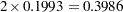## Example 60.1 Cochran-Armitage Test with Permutation Resampling

This example, from Keith Soper at Merck, illustrates the exact permutation Cochran-Armitage test carried out on permutation resamples. In the following data set, each observation represents an animal. The binary variables S1 and S2 indicate two tumor types, with 0s indicating no tumor (failure) and 1 indicating a tumor (success); note that they have perfect negative association. The grouping variable is Dose.

```data a;
input S1 S2 Dose @@;
datalines;
0 1 1   1 0 1   0 1 1
0 1 1   0 1 1   1 0 1
1 0 2   1 0 2   0 1 2
1 0 2   0 1 2   1 0 2
1 0 3   1 0 3   1 0 3
0 1 3   0 1 3   1 0 3
;
```
```proc multtest data=a permutation nsample=10000 seed=36607 outperm=pmt;
test ca(S1 S2 / permutation=10 uppertailed);
class Dose;
contrast 'CA Linear Trend' 0 1 2;
run;
proc print data=pmt;
run;
```

The PROC MULTTEST statement requests 10,000 permutation resamples. The OUTPERM= option creates an output SAS data set pmt used for the exact permutation distribution computed for the CA test.

The TEST statement specifies an upper-tailed Cochran-Armitage linear trend test for S1 and S2. The cutoff for exact permutation calculations is 10, as specified with the PERMUTATION= option in the TEST statement. Since S1 and S2 have 10 and 8 successes, respectively, PROC MULTTEST uses exact permutation distributions to compute the p-values for both variables.

The groups for the CA test are the levels of Dose from the CLASS statement. The trend coefficients applied to these groups are 0, 1, and 2, respectively, as specified in the CONTRAST statement.

Finally, the PROC PRINT statement displays the SAS data set pmt, which contains the permutation distributions.

The results from this analysis are displayed in Output 60.1.1 through Output 60.1.5. You should check the "Model Information" table to verify that the analysis specifications are correct.

Output 60.1.1 Cochran-Armitage Test with Permutation Resampling
The Multtest Procedure

Model Information
Test for discrete variables Cochran-Armitage
Exact permutation distribution used Everywhere
Tails for discrete tests Upper-tailed
Strata weights None
Number of resamples 10000
Seed 36607

The label and coefficients from the CONTRAST statement are shown in Output 60.1.2.

Output 60.1.2 Contrast Coefficients
Contrast Coefficients
Contrast Dose
1 2 3
CA Linear Trend 0 1 2

Output 60.1.3 displays summary statistics for the two test variables, S1 and S2. The Count column lists the number of successes for each level of the CLASS variable, Dose. The NumObs column lists the sample size, and the Percent column lists the percentage of successes in the sample.

Output 60.1.3 Summary Statistics
Discrete Variable Tabulations
Variable Dose Count NumObs Percent
S1 1 2 6 33.33
S1 2 4 6 66.67
S1 3 4 6 66.67
S2 1 4 6 66.67
S2 2 2 6 33.33
S2 3 2 6 33.33

The Raw column in Output 60.1.4 contains the p-values from the CA test, and the Permutation column contains the permutation-adjusted p-values.

Output 60.1.4 Resulting p-Values
p-Values
Variable Contrast Raw Permutation
S1 CA Linear Trend 0.1993 0.4009
S2 CA Linear Trend 0.9220 1.0000

This table shows that, for S1, the adjusted p-value is approximately twice the raw p-value. In fact, resamples with small (large) p-values for S1 have large (small) p-values for S2 due to the perfect negative association of the variables, and hence the permutation-adjusted p-value for S1 should be; the difference is due to resampling error. For the same reason, since the raw p-value for S2 is 0.9220, the adjusted p-value equals 1. The permutation p-values for S1 and S2 also happen to be the Bonferroni-adjusted p-values for this example.

The OUTPERM= data set is displayed in Output 60.1.5, which contains the exact permutation distributions for S1 and S2 in terms of cumulative probabilities.

Output 60.1.5 Exact Permutation Distribution
Obs _contrast_ _var_ _value_ upper_p
1 CA Linear Trend S1 0 1.00000
2 CA Linear Trend S1 1 1.00000
3 CA Linear Trend S1 2 1.00000
4 CA Linear Trend S1 3 1.00000
5 CA Linear Trend S1 4 1.00000
6 CA Linear Trend S1 5 0.99966
7 CA Linear Trend S1 6 0.99609
8 CA Linear Trend S1 7 0.97827
9 CA Linear Trend S1 8 0.92205
10 CA Linear Trend S1 9 0.80070
11 CA Linear Trend S1 10 0.61011
12 CA Linear Trend S1 11 0.38989
13 CA Linear Trend S1 12 0.19930
14 CA Linear Trend S1 13 0.07795
15 CA Linear Trend S1 14 0.02173
16 CA Linear Trend S1 15 0.00391
17 CA Linear Trend S1 16 0.00034
18 CA Linear Trend S1 17 0.00000
19 CA Linear Trend S1 18 0.00000
20 CA Linear Trend S1 19 0.00000
21 CA Linear Trend S1 20 0.00000
22 CA Linear Trend S2 0 1.00000
23 CA Linear Trend S2 1 1.00000
24 CA Linear Trend S2 2 1.00000
25 CA Linear Trend S2 3 0.99966
26 CA Linear Trend S2 4 0.99609
27 CA Linear Trend S2 5 0.97827
28 CA Linear Trend S2 6 0.92205
29 CA Linear Trend S2 7 0.80070
30 CA Linear Trend S2 8 0.61011
31 CA Linear Trend S2 9 0.38989
32 CA Linear Trend S2 10 0.19930
33 CA Linear Trend S2 11 0.07795
34 CA Linear Trend S2 12 0.02173
35 CA Linear Trend S2 13 0.00391
36 CA Linear Trend S2 14 0.00034
37 CA Linear Trend S2 15 0.00000
38 CA Linear Trend S2 16 0.00000